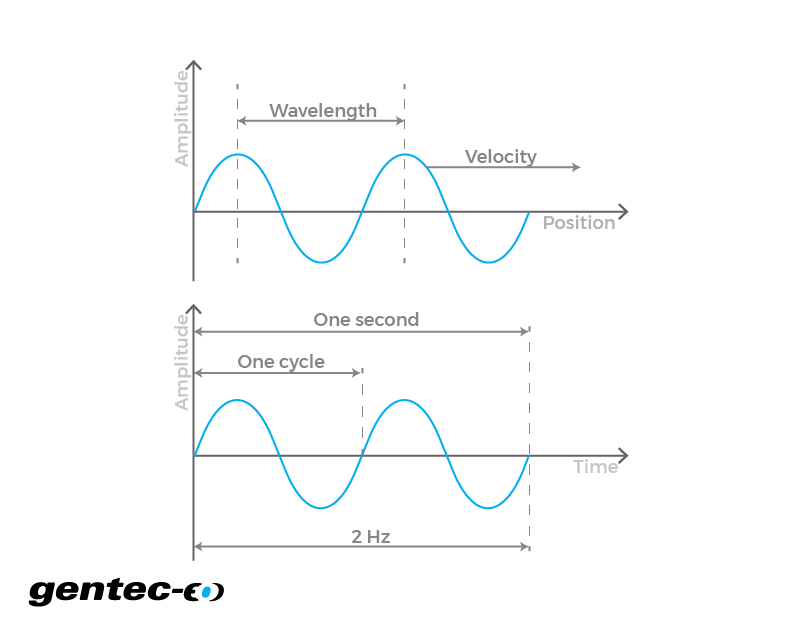EN

# Wavelength and frequency

Easily convert your laser wavelength to frequency and laser frequency to wavelength with this free online calculator.

- m

- Hz

## How does the laser wavelength and frequency converter work?

For a given wave, the relationship between frequency and wavelength is linked by its velocity. In the case of an electromagnetic wave in vacuum, its propagation velocity is equal to c, the speed of light, which is defined as 299 792 458 m/s in SI. The frequency corresponds to the number of times a wave/particle vibrates during a certain time period, or how many times it comes back to the same state per time unit. It's usually measured in Hertz (1/s). The wavelength is the distance in space between those vibrations, or the distance that separates the two closest points where the wave is in the same state. It is usually measured in meters.

Click to
enlarge## The formula of wavelength and frequency

In this wavelength and frequency calculator, we assumed that the conversion was meant for a monochromatic electromagnetic wave in vacuum. So the velocity of the wave was presumed equal to c and the wavelength and frequency each possesses discrete values. You can use the equation displayed here to convert frequency to wavelength.

$$\text{Frequency}(Hz) = \frac{\text{Velocity}(m/s)}{\text{Wavelength}(m)}$$

Right-click
to copy